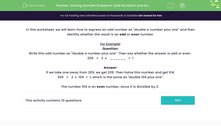# Solving Number Problems: Odd Numbers and Doubling

In this worksheet, students express an odd number as 2n+1 and then asking whether n is odd or even.Key stage:  KS 2

Curriculum topic:   Algebra

Curriculum subtopic:   Express Problems Algebraically

Difficulty level:#### Worksheet Overview

In this worksheet, we will learn how to express an odd number as "double a number plus one" and then identify whether the result is an odd or even number.

For Example:

Question:
Write this odd number as "double a number plus one". Then say whether the answer is odd or even.

209   =   2  x    ______   +  1

If we take one away from 209, we get 208. Then halve this number and get 104.

209   =   2  x  104  +  1, which is the same as "double 104 plus one".

The number 104 is an even number, since it is divisible by 2.

### What is EdPlace?

We're your National Curriculum aligned online education content provider helping each child succeed in English, maths and science from year 1 to GCSE. With an EdPlace account you’ll be able to track and measure progress, helping each child achieve their best. We build confidence and attainment by personalising each child’s learning at a level that suits them.

Get started